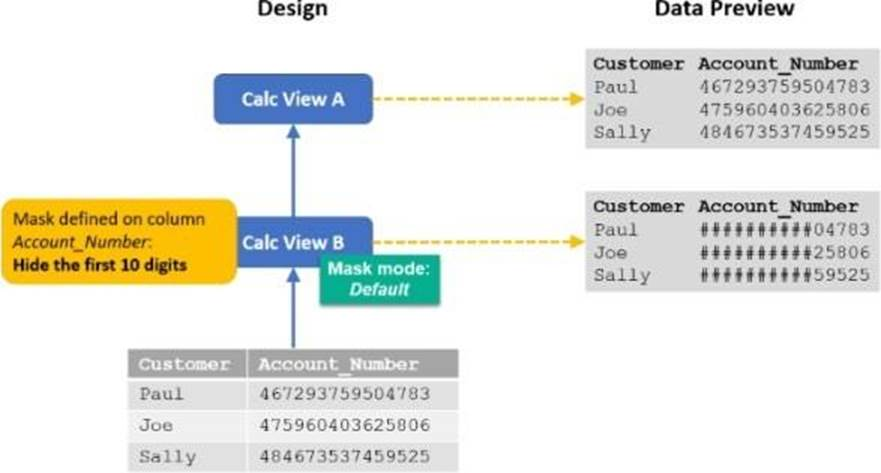## SAP HANA Cloud Modeling C_HCMOD_02 Dumps Questions

The best tips to pass SAP C_HCMOD_02 exam is to use C_HCMOD_02 Dumps Questions. FreeTestShare SAP HANA Cloud Modeling C_HCMOD_02 Dumps Questions allow you to practice the types of questions that may occur on the exam. C_HCMOD_02 Dumps Questions are exactly the right study tools that contain actual questions and answers to help you pass the SAP C_HCMOD_02 exam on the first try!  By practicing with our C_HCMOD_02 Dumps Questions, you will be able to easily answer all the questions.

Before you buy, take a free C_HCMOD_02 practice test to assess your skills!

Page 1 of 2

1. In a calculation view, why would you implement an SQL expression? Note: There are 3 correct answers to this question.

2. In a calculation view, your table function node executes a table function that requires input parameters .

How can you fill the input parameters of the table function? Note: There are 3 correct answers to this question.

3. You create a table function to remove historic records, sum the current total weekly working hours for each employee, and update the personnel table with the results. The deployment of the table function fails.

Which of the following could be a valid reason?

4. 1. What is the SQL keyword used to process input parameters defined in a calculation view?

5. Which database features are typically NOT required by analytical applications that run on SAP HANA Cloud? Note: There are 2 correct answers to this question.

6. Which project structure object corresponds to a unique HDI container?

7. Why would you set the "Ignore multiple outputs for filters" property in a calculation view?

8. Which of the following are standard options provided to define analytic privileges? Note: There are 3 correct answers to this question.

9. You are deploying a new calculation view, A, that uses calculation view B, as shown in the diagram.When you preview calculation view A, the account number is not masked.

What could be the reason?

10. You set the Null Handling property for an attribute but do not set a default value.

What is displayed when null values are found in a column of data type NVARCHAR?